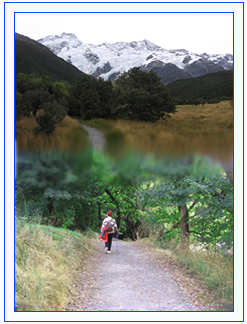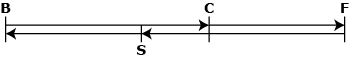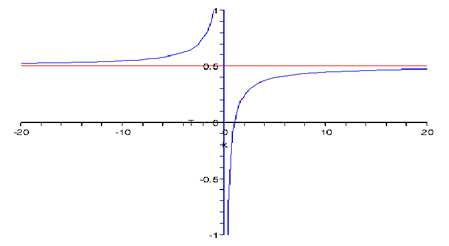# Bush walkingIt is not uncommon for companions who enjoy bush walking to differ in fitness and energy. On tracks that lead out and back they will often walk together for a time at the pace of the slower walker – until s/he indicates an intention to turn around and return to base.

The faster walker has the choice of following the same action, but alternatively may decide to push on for a time at her/his faster pace before also returning. Especially if the opportunity to travel further and faster is appreciated, the faster walker will want to go as far as possible.

However they will not want their companion to have to wait around at the end of the walk for them to return.

So an interesting problem emerges. When the slower walker starts the return trip, for how much extra time should the faster walker travel on the outward path before turning for home – so that both will arrive at the starting point at the same time?

The Source: Walking track at (Aoraki - Mount Cook)

#### Basic problemBF and FB are outward and return paths along the same track. Assume that after parting both walkers maintain their respective average walking speeds.

• B = starting point (Base)
• C = point that walkers reach together after walking for time (t) at the speed (V) of the slower walker.

Slower walker (s) now starts to return while the faster walker (f) continues on at speed (kV) where k > 1.

• F = point at which ‘f’ turns back after travelling for an additional time (T) at the faster speed.
• S is the point reached by s on the homeward path when f turns for home at F.

#### Walkers to arrive at B togetherBC = Vt; CF = (kV)T; CS = VT

So FB/kV = SB/V

FB = BC + CF = Vt +kVT

SB = BC – CS  = Vt –VT

Hence V(t + kT)/kV = V(t–T)/V

So             (t + kT)/k = (t–T)

2kT = (k–1)t

T = [(k–1)/2k]t

So it is sufficient to know the time from the start of the walk to the separation point (t), and the relative walking speeds (k) of the two individuals.

Check: k=1 gives T = 0  (both walkers turn together if they walk at the same pace, as should happen).

Example: Walkers stay together for an hour: t =1 so T = (k–1)/2k

Suppose k =2: T = ¼ (‘f’ should continue on for 15 minutes) etcRelevant section of  k – T graph (k ≥1)

#### Wings on the heels: investigate limiting behaviour

Here we assume that ‘f’ can travel at any multiple of the speed of ‘s’ – k has no upper bound.

Taking again the case where the walkers stay together for an hour (t = 1) we have T = (k-1)/2k = ½ - 1/2k

As k → ∞, T → ½ (see graph).

If T = ½ there is nothing ‘f’ can do to catch up as ‘s’ is already back at the base when ‘f’ reaches C on the return trip.

Now suppose ‘f’ turns after 27 min (T = 0.45)

(Then ‘s’ is 33 minutes from base)

Need  ½ - 1/2k   = 0.45

1/2k   = 0.05

2k = 20

k = 10

If T = 0.4833 (29 min) k = 29.94

If T = 0.499722 (29 min 59 sec) k= 1799.998

Nominate any value of time (T) less than 0.5, and we can always find a speed multiple (k) that will enable ‘s’ and ‘f’ to finish together.

#### Further refinement

It is more realistic in practical terms for ‘f’ to aim to arrive at base so that ‘s’ doesn’t have to wait ‘too long’ – that is allowing a time window ‘w’.

Then we have 0 < (t + kT)/k – (t–T) < w

0 < [2kT – t(k – 1)]/k < w

t(k– 1)/2k < T < [k(t+w) – t]/2k

For example if k =2, t =1, w = 0.2 (12 minutes) we have 0.25 < T < 0.35

So ‘f’ should continue on for a time between 15 and 21 minutes.

* k > 0 from its real world property which is important in manipulating the inequalities.

#### Mathematical content accessed in problem

d = v x t  | proportion | ratio| equations | rational functions - hyperbolas | graphical technology | estimation | inequalities | limits

#### ResourcesGo to the Starting Points Forum to join the discussion or find more Starting Points